# Arrays

9. Aug 2018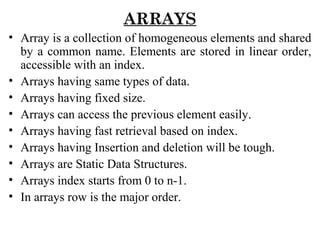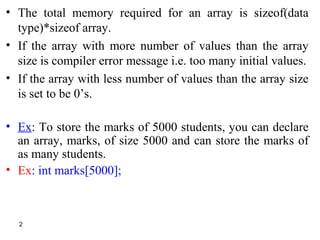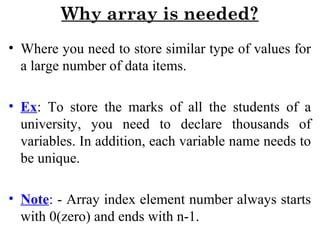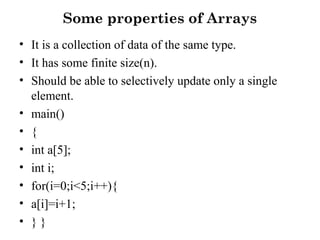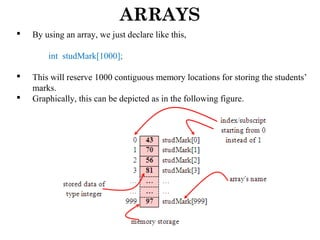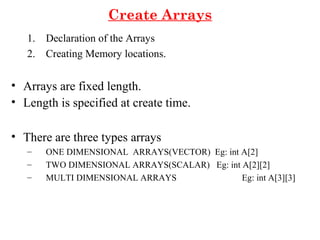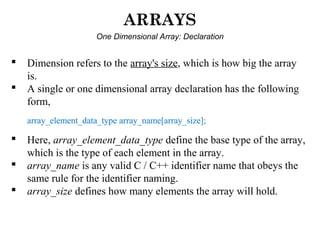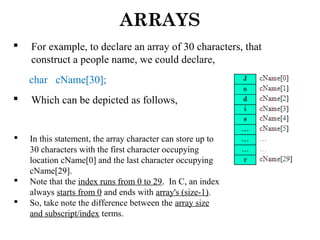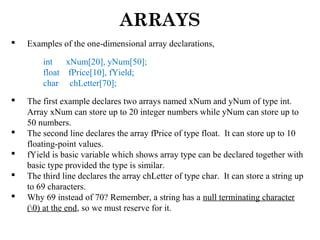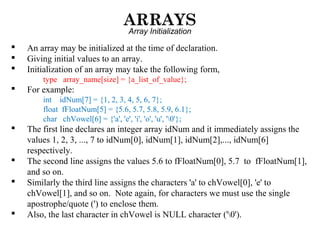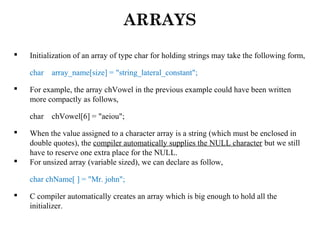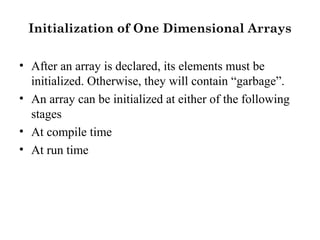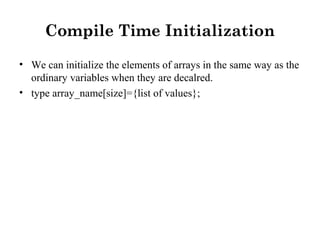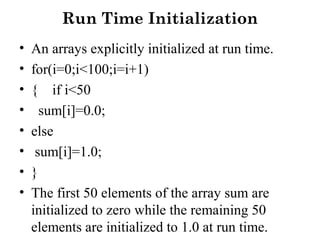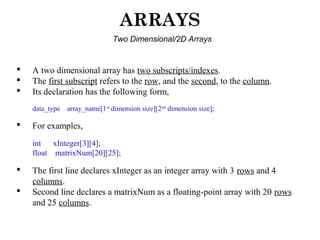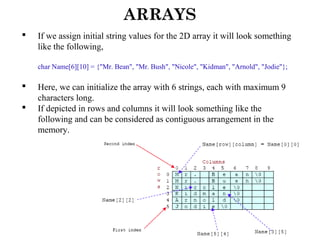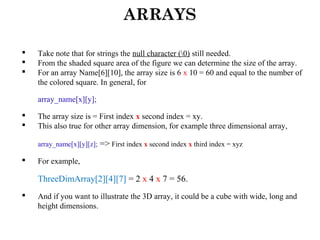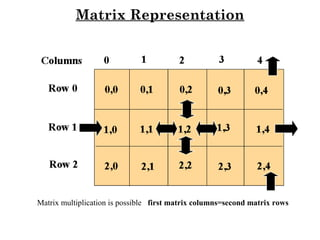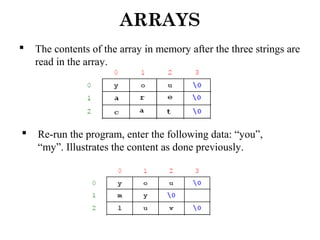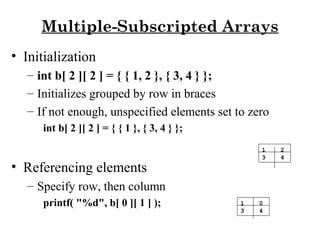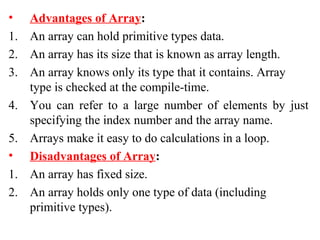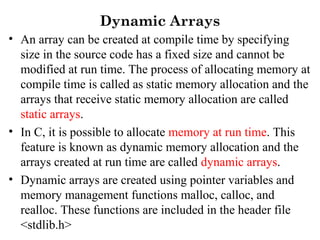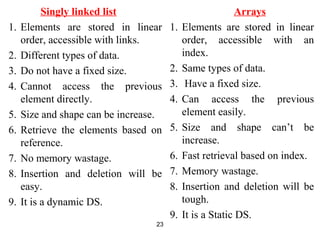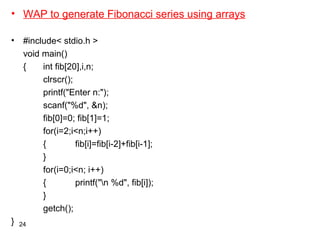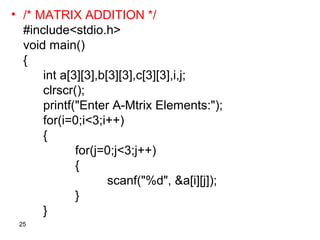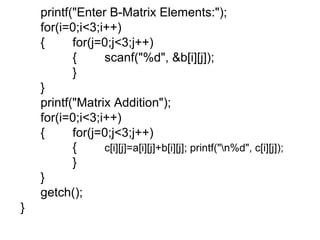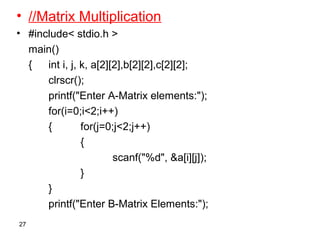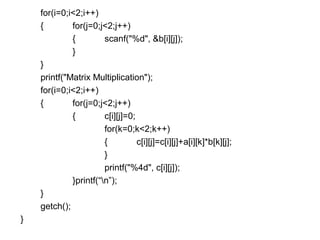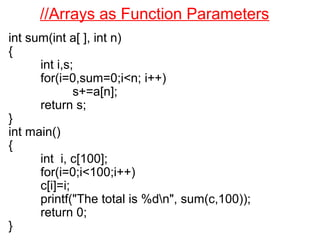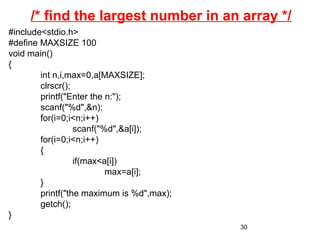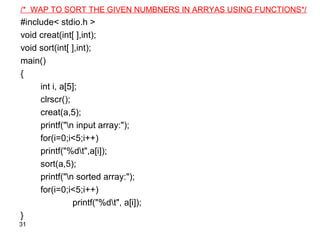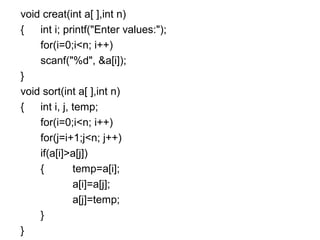1 von 32

### Arrays

• 1. ARRAYS • Array is a collection of homogeneous elements and shared by a common name. Elements are stored in linear order, accessible with an index. • Arrays having same types of data. • Arrays having fixed size. • Arrays can access the previous element easily. • Arrays having fast retrieval based on index. • Arrays having Insertion and deletion will be tough. • Arrays are Static Data Structures. • Arrays index starts from 0 to n-1. • In arrays row is the major order.
• 2. • The total memory required for an array is sizeof(data type)*sizeof array. • If the array with more number of values than the array size is compiler error message i.e. too many initial values. • If the array with less number of values than the array size is set to be 0’s. • Ex: To store the marks of 5000 students, you can declare an array, marks, of size 5000 and can store the marks of as many students. • Ex: int marks; 2
• 3. Why array is needed? • Where you need to store similar type of values for a large number of data items. • Ex: To store the marks of all the students of a university, you need to declare thousands of variables. In addition, each variable name needs to be unique. • Note: - Array index element number always starts with 0(zero) and ends with n-1.
• 4. Some properties of Arrays • It is a collection of data of the same type. • It has some finite size(n). • Should be able to selectively update only a single element. • main() • { • int a; • int i; • for(i=0;i<5;i++){ • a[i]=i+1; • } }
• 5. ARRAYS  By using an array, we just declare like this, int studMark;  This will reserve 1000 contiguous memory locations for storing the students’ marks.  Graphically, this can be depicted as in the following figure.
• 6. Create Arrays 1. Declaration of the Arrays 2. Creating Memory locations. • Arrays are fixed length. • Length is specified at create time. • There are three types arrays – ONE DIMENSIONAL ARRAYS(VECTOR) Eg: int A – TWO DIMENSIONAL ARRAYS(SCALAR) Eg: int A – MULTI DIMENSIONAL ARRAYS Eg: int A
• 7. ARRAYS One Dimensional Array: Declaration  Dimension refers to the array's size, which is how big the array is.  A single or one dimensional array declaration has the following form, array_element_data_type array_name[array_size];  Here, array_element_data_type define the base type of the array, which is the type of each element in the array.  array_name is any valid C / C++ identifier name that obeys the same rule for the identifier naming.  array_size defines how many elements the array will hold.
• 8. ARRAYS  For example, to declare an array of 30 characters, that construct a people name, we could declare, char cName;  Which can be depicted as follows,  In this statement, the array character can store up to 30 characters with the first character occupying location cName and the last character occupying cName.  Note that the index runs from 0 to 29. In C, an index always starts from 0 and ends with array's (size-1).  So, take note the difference between the array size and subscript/index terms.
• 9. ARRAYS  Examples of the one-dimensional array declarations, int xNum, yNum; float fPrice, fYield; char chLetter;  The first example declares two arrays named xNum and yNum of type int. Array xNum can store up to 20 integer numbers while yNum can store up to 50 numbers.  The second line declares the array fPrice of type float. It can store up to 10 floating-point values.  fYield is basic variable which shows array type can be declared together with basic type provided the type is similar.  The third line declares the array chLetter of type char. It can store a string up to 69 characters.  Why 69 instead of 70? Remember, a string has a null terminating character (0) at the end, so we must reserve for it.
• 10. ARRAYS Array Initialization  An array may be initialized at the time of declaration.  Giving initial values to an array.  Initialization of an array may take the following form, type array_name[size] = {a_list_of_value};  For example: int idNum = {1, 2, 3, 4, 5, 6, 7}; float fFloatNum = {5.6, 5.7, 5.8, 5.9, 6.1}; char chVowel = {'a', 'e', 'i', 'o', 'u', '0'};  The first line declares an integer array idNum and it immediately assigns the values 1, 2, 3, ..., 7 to idNum, idNum, idNum,..., idNum respectively.  The second line assigns the values 5.6 to fFloatNum, 5.7 to fFloatNum, and so on.  Similarly the third line assigns the characters 'a' to chVowel, 'e' to chVowel, and so on. Note again, for characters we must use the single apostrophe/quote (') to enclose them.  Also, the last character in chVowel is NULL character ('0').
• 11. ARRAYS  Initialization of an array of type char for holding strings may take the following form, char array_name[size] = "string_lateral_constant";  For example, the array chVowel in the previous example could have been written more compactly as follows, char chVowel = "aeiou";  When the value assigned to a character array is a string (which must be enclosed in double quotes), the compiler automatically supplies the NULL character but we still have to reserve one extra place for the NULL.  For unsized array (variable sized), we can declare as follow, char chName[ ] = "Mr. john";  C compiler automatically creates an array which is big enough to hold all the initializer.
• 12. Initialization of One Dimensional Arrays • After an array is declared, its elements must be initialized. Otherwise, they will contain “garbage”. • An array can be initialized at either of the following stages • At compile time • At run time
• 13. Compile Time Initialization • We can initialize the elements of arrays in the same way as the ordinary variables when they are decalred. • type array_name[size]={list of values};
• 14. Run Time Initialization • An arrays explicitly initialized at run time. • for(i=0;i<100;i=i+1) • { if i<50 • sum[i]=0.0; • else • sum[i]=1.0; • } • The first 50 elements of the array sum are initialized to zero while the remaining 50 elements are initialized to 1.0 at run time.
• 15. ARRAYS Two Dimensional/2D Arrays  A two dimensional array has two subscripts/indexes.  The first subscript refers to the row, and the second, to the column.  Its declaration has the following form, data_type array_name[1st dimension size][2nd dimension size];  For examples, int xInteger; float matrixNum;  The first line declares xInteger as an integer array with 3 rows and 4 columns.  Second line declares a matrixNum as a floating-point array with 20 rows and 25 columns.
• 16. ARRAYS  If we assign initial string values for the 2D array it will look something like the following, char Name = {"Mr. Bean", "Mr. Bush", "Nicole", "Kidman", "Arnold", "Jodie"};  Here, we can initialize the array with 6 strings, each with maximum 9 characters long.  If depicted in rows and columns it will look something like the following and can be considered as contiguous arrangement in the memory.
• 17. ARRAYS  Take note that for strings the null character (0) still needed.  From the shaded square area of the figure we can determine the size of the array.  For an array Name, the array size is 6 x 10 = 60 and equal to the number of the colored square. In general, for array_name[x][y];  The array size is = First index x second index = xy.  This also true for other array dimension, for example three dimensional array, array_name[x][y][z]; => First index x second index x third index = xyz  For example, ThreeDimArray = 2 x 4 x 7 = 56.  And if you want to illustrate the 3D array, it could be a cube with wide, long and height dimensions.
• 18. Matrix Representation Matrix multiplication is possible first matrix columns=second matrix rows
• 19. ARRAYS  The contents of the array in memory after the three strings are read in the array.  Re-run the program, enter the following data: “you”, “my”. Illustrates the content as done previously.
• 20. Multiple-Subscripted Arrays • Initialization – int b[ 2 ][ 2 ] = { { 1, 2 }, { 3, 4 } }; – Initializes grouped by row in braces – If not enough, unspecified elements set to zero int b[ 2 ][ 2 ] = { { 1 }, { 3, 4 } }; • Referencing elements – Specify row, then column printf( "%d", b[ 0 ][ 1 ] );   1 2 3 4 1 0 3 4
• 21. • Advantages of Array: 1. An array can hold primitive types data. 2. An array has its size that is known as array length. 3. An array knows only its type that it contains. Array type is checked at the compile-time. 4. You can refer to a large number of elements by just specifying the index number and the array name. 5. Arrays make it easy to do calculations in a loop. • Disadvantages of Array: 1. An array has fixed size. 2. An array holds only one type of data (including primitive types).
• 22. Dynamic Arrays • An array can be created at compile time by specifying size in the source code has a fixed size and cannot be modified at run time. The process of allocating memory at compile time is called as static memory allocation and the arrays that receive static memory allocation are called static arrays. • In C, it is possible to allocate memory at run time. This feature is known as dynamic memory allocation and the arrays created at run time are called dynamic arrays. • Dynamic arrays are created using pointer variables and memory management functions malloc, calloc, and realloc. These functions are included in the header file <stdlib.h>
• 23. Singly linked list 1. Elements are stored in linear order, accessible with links. 2. Different types of data. 3. Do not have a fixed size. 4. Cannot access the previous element directly. 5. Size and shape can be increase. 6. Retrieve the elements based on reference. 7. No memory wastage. 8. Insertion and deletion will be easy. 9. It is a dynamic DS. Arrays 1. Elements are stored in linear order, accessible with an index. 2. Same types of data. 3. Have a fixed size. 4. Can access the previous element easily. 5. Size and shape can’t be increase. 6. Fast retrieval based on index. 7. Memory wastage. 8. Insertion and deletion will be tough. 9. It is a Static DS. 23
• 24. • WAP to generate Fibonacci series using arrays • #include< stdio.h > void main() { int fib,i,n; clrscr(); printf("Enter n:"); scanf("%d", &n); fib=0; fib=1; for(i=2;i<n;i++) { fib[i]=fib[i-2]+fib[i-1]; } for(i=0;i<n; i++) { printf("n %d", fib[i]); } getch(); } 24
• 25. 25 • /* MATRIX ADDITION */ #include<stdio.h> void main() { int a,b,c,i,j; clrscr(); printf("Enter A-Mtrix Elements:"); for(i=0;i<3;i++) { for(j=0;j<3;j++) { scanf("%d", &a[i][j]); } }
• 26. printf("Enter B-Matrix Elements:"); for(i=0;i<3;i++) { for(j=0;j<3;j++) { scanf("%d", &b[i][j]); } } printf("Matrix Addition"); for(i=0;i<3;i++) { for(j=0;j<3;j++) { c[i][j]=a[i][j]+b[i][j]; printf("n%d", c[i][j]); } } getch(); }
• 27. • //Matrix Multiplication • #include< stdio.h > main() { int i, j, k, a,b,c; clrscr(); printf("Enter A-Matrix elements:"); for(i=0;i<2;i++) { for(j=0;j<2;j++) { scanf("%d", &a[i][j]); } } printf("Enter B-Matrix Elements:"); 27
• 28. for(i=0;i<2;i++) { for(j=0;j<2;j++) { scanf("%d", &b[i][j]); } } printf("Matrix Multiplication"); for(i=0;i<2;i++) { for(j=0;j<2;j++) { c[i][j]=0; for(k=0;k<2;k++) { c[i][j]=c[i][j]+a[i][k]*b[k][j]; } printf("%4d", c[i][j]); }printf(“n”); } getch(); }
• 29. //Arrays as Function Parameters int sum(int a[ ], int n) { int i,s; for(i=0,sum=0;i<n; i++) s+=a[n]; return s; } int main() { int i, c; for(i=0;i<100;i++) c[i]=i; printf("The total is %dn", sum(c,100)); return 0; }
• 30. 30 /* find the largest number in an array */ #include<stdio.h> #define MAXSIZE 100 void main() { int n,i,max=0,a[MAXSIZE]; clrscr(); printf("Enter the n:"); scanf("%d",&n); for(i=0;i<n;i++) scanf("%d",&a[i]); for(i=0;i<n;i++) { if(max<a[i]) max=a[i]; } printf("the maximum is %d",max); getch(); }
• 31. /* WAP TO SORT THE GIVEN NUMBNERS IN ARRYAS USING FUNCTIONS*/ #include< stdio.h > void creat(int[ ],int); void sort(int[ ],int); main() { int i, a; clrscr(); creat(a,5); printf("n input array:"); for(i=0;i<5;i++) printf("%dt",a[i]); sort(a,5); printf("n sorted array:"); for(i=0;i<5;i++) printf("%dt", a[i]); } 31
• 32. void creat(int a[ ],int n) { int i; printf("Enter values:"); for(i=0;i<n; i++) scanf("%d", &a[i]); } void sort(int a[ ],int n) { int i, j, temp; for(i=0;i<n; i++) for(j=i+1;j<n; j++) if(a[i]>a[j]) { temp=a[i]; a[i]=a[j]; a[j]=temp; } }